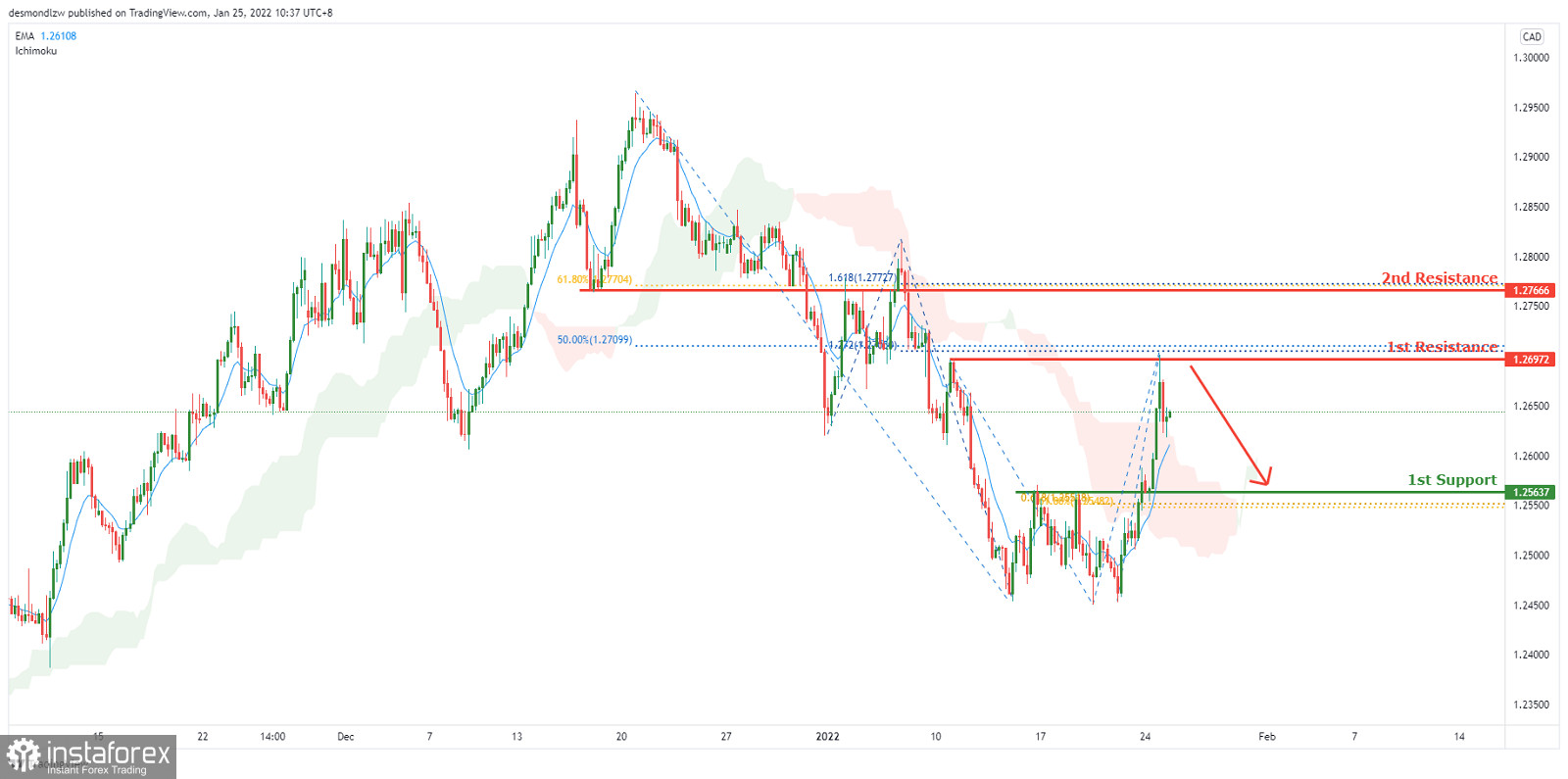# FX.co ★ USDCAD, Bearish Pressure | 25th Jan 2022

Forex Analysis:::2022-01-25T05:08:56

## USDCAD, Bearish Pressure | 25th Jan 2022On the H4 chart price is near resistance of 1.26972 which is also 50% Fibonacci retracement and 127.2% Fibonacci projection. Price can potentially dip to the 1st support level of 1.25637 which is also 61.8% Fibonacci retracement and 61.8% Fibonacci projection. Our bearish bias is supported by the Ichimoku cloud indicator. Alternatively price can go to the 2nd resistance level of 1.27666 which is 61.8% Fibonacci retracement and 161.8% Fibonacci projection.

Entry:1.26972

Reason for Entry:

Horizontal overlap resistance , 50% Fibonacci retracement and 127.2% Fibonacci projection

Take Profit: 1.24604

Reason for Take Profit:

Horizontal overlap support , 61.8% Fibonacci projection and 61.8% Fibonacci retracement

Stop Loss: 1.27666

Reason for Stop Loss:

Horizontal overlap resistance and 161.8% Fibonacci projection and 61.8% Fibonacci retracement

Analyst InstaForex